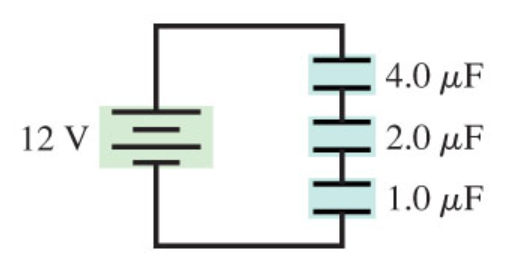# Problem: (a) What is the equivalent capacitance for the circuit of the figure?(b) What is the charge of the capacitor 4.0 μF?(c) What is the charge of the capacitor 2.0 μF?(d) What is the charge of the capacitor 1.0 μF?

###### FREE Expert Solution

For capacitors in series, the equivalent capacitance is:

$\overline{)\frac{\mathbf{1}}{{\mathbf{C}}_{\mathbf{e}\mathbf{q}}}{\mathbf{=}}\frac{\mathbf{1}}{{\mathbf{C}}_{\mathbf{1}}}{\mathbf{+}}\frac{\mathbf{1}}{{\mathbf{C}}_{\mathbf{2}}}{\mathbf{+}}{\mathbf{.}}{\mathbf{.}}{\mathbf{.}}{\mathbf{+}}\frac{\mathbf{1}}{{\mathbf{C}}_{\mathbf{n}}}}$

Charge:

$\overline{){\mathbf{Q}}{\mathbf{=}}{{\mathbf{C}}}_{{\mathbf{eq}}}{\mathbf{V}}}$

(a)

Equivalent capacitor:

1/Ceq = 1/4.0 + 1/2.0 + 1/1.0 = 7/4

95% (345 ratings)###### Problem Details

(a) What is the equivalent capacitance for the circuit of the figure?
(b) What is the charge of the capacitor 4.0 μF?
(c) What is the charge of the capacitor 2.0 μF?
(d) What is the charge of the capacitor 1.0 μF?